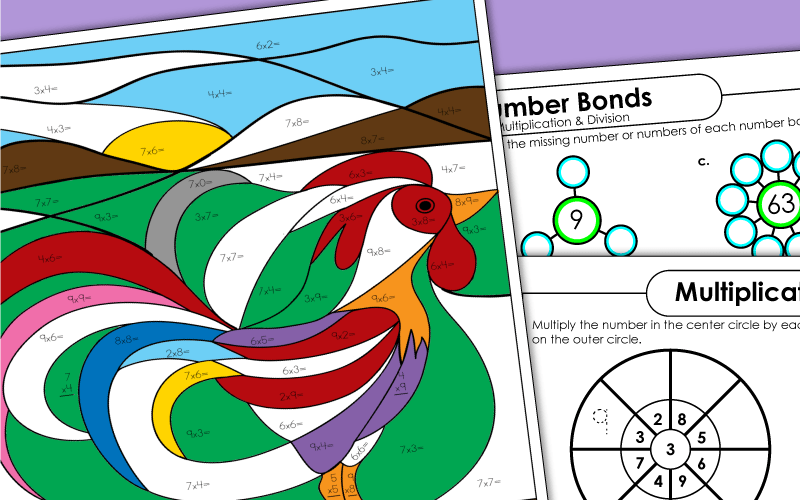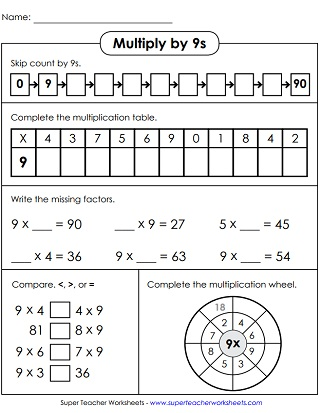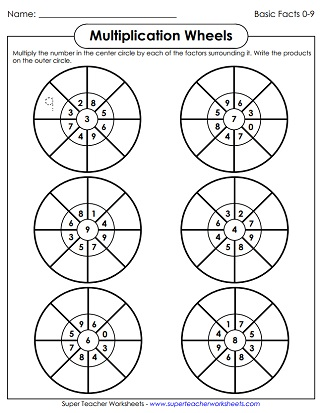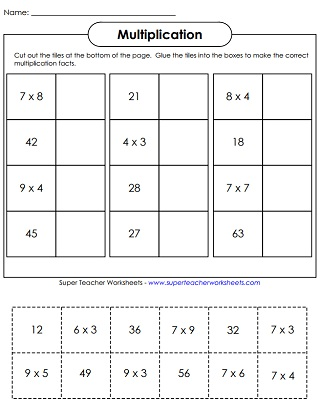# Multiplying by 9s

Use these printables to help your students master basic multiplication facts with 9 as a factor. Also find sheets with all facts up to 9s.## Multiplication by9s Only

Learn how to quickly and easily multiply by nines using the fingers on your hands!
On this printable, students begin by skip counting from 0 to 90 by 9s. Then they fill in a scrambled multiplication table with 9s. After that, they compare numbers and complete a magic multiplication wheel.
Solve for each multiplication fact with a factor of 9. Then color according to the key. The finished picture is a friendly, brown dog.
Have students practice multiplying by 9 with these task cards. They can be used for various games and activities, such as scavenger hunts and peer practice.
One way to play with this puzzle is to race to make a match. Give two or more students a set of multiplication fact and answer cards. Have them race to see who can correctly match all of the cards first.
Children will multiply by 9s to complete this printable multiplication activity.
Cut out the pattern and use it to make an origami cootie catcher with basic facts with 9 as a factor.
Use these cards to review multiplication facts with 9 as a factor. Includes flash cards, a self-test and a sorting mat.
Cut out the apple and math fact strips. Weave the strips through the apple. Review multiplication facts with 8 as a factor. For best results, print this file on thick card paper.

## Skip Count by 9s

Skip count by 9s. Then answer the related word problems and critical thinking questions.
Use the number line as an aid to skip-count by nines. The number lines go up to 99.
Count by 9s and write the missing numbers on the penguins' bellies. Up to 225.
Count by 9s and write the missing numbers on the colorful balloons.

## All Facts0s through 9s

This practice worksheet has facts with factors through 9s, up to 9x10.
This page has problems all basic multiplication facts, 0 through 9, up to 9x12.
This is a timed assessment with 50 questions on it. Check to see how many your students can answer quickly and accurately. Facts through 12, up to 9x12.
These multiplication wheels contain all facts through 9s, up to 9x9.
Cut out the squares at the bottom. Match the facts with the products. Then glue them onto the page.
Determine whether each problem in correct or incorrect. If it's correct, color the box green. If it's not correct, write the correct answer.
Look carefully at each domino and multiply both halves together. Up to 9x9.
This bingo game has 30 different boards and a set of calling cards with facts up to 9x9.
This memory match game has facts up through the 9s.
Unscramble the numbers to make complete multiplication facts. (Does not include any facts with 10s, 11s, or 12s.)
This is a drill worksheet with many facts up to 9x9. Color the boxes according to the key.
This is a printable space-themed board game. The question cards have multiplication facts up to 9x9.
Complete the math problems and color according to the key to make a picture of a sea monster.
In this mystery picture, students will complete the facts to create a hot air balloon.
Cock-a-doodle-doo! Here's a rooster mystery picture that kids will love.
Multiplication Basic Facts: 0-10

Here you'll find lots more multiplication worksheets, games, and activities for all basic facts up to 10x10.

Multiplication/Division
Fact Families

This page has lots of worksheets with multiplication and division fact families.

Multiplication by 3s

Multiplication by 4s

These worksheets focus specifically on the 4s times tables.

Multiplication by 5s

Here are the activities for learning multiplication facts from 5x0 up to 5x12.

Multiplication by 6s

Multiplication by 7s

Basic facts with factors of 7 are the focus on these printable learning resources.

Multiplication by 8s

Practice multiplying by the number 8. Includes mystery pictures, skip counting lessons, and more.

## Worksheet ImagesMy Account
Site Information